Publicité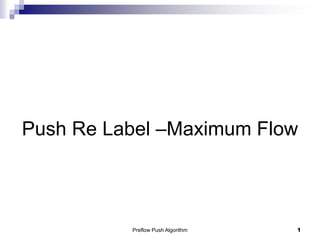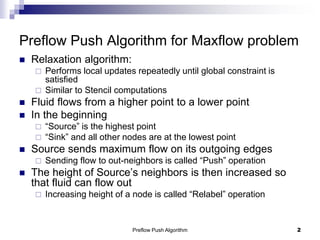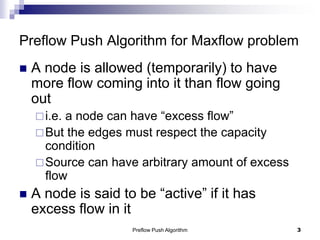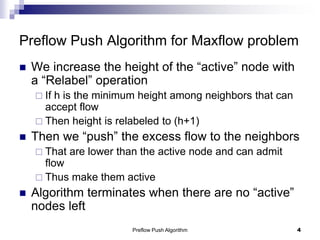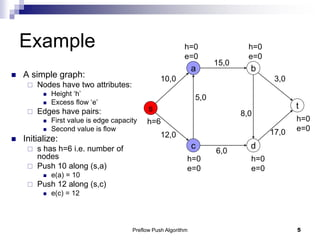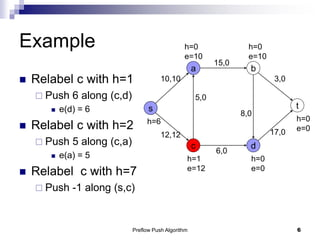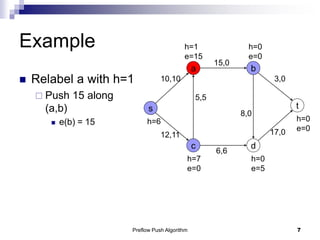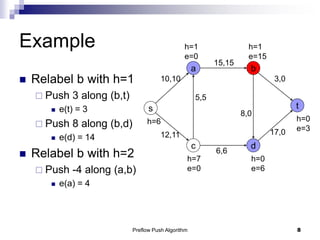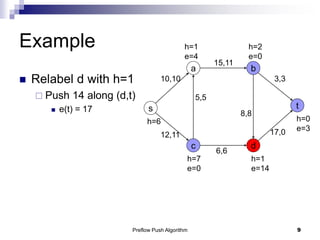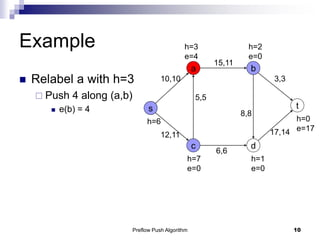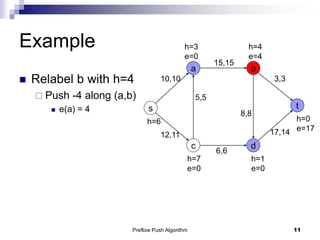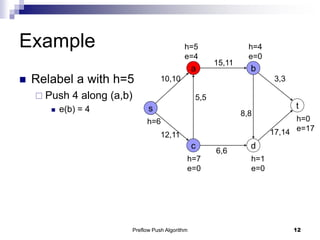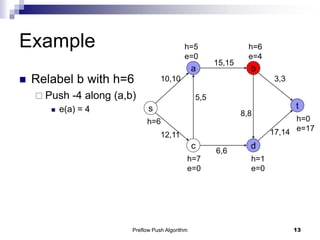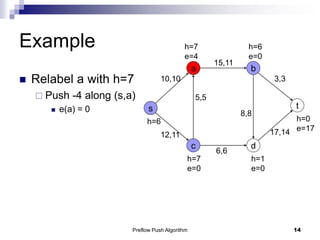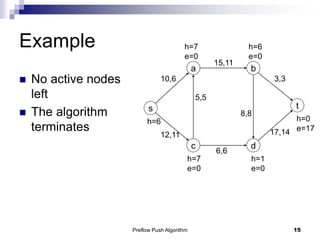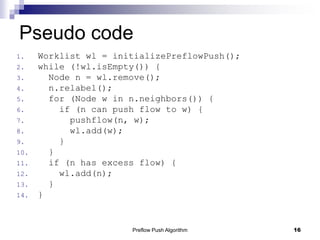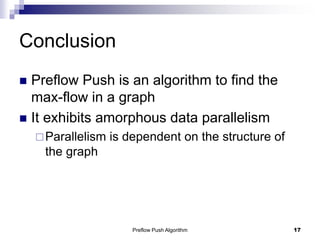1 sur 17
Publicité

### preflowpush.ppt

1. Push Re Label –Maximum Flow Preflow Push Algorithm 1
2. Preflow Push Algorithm 2 Preflow Push Algorithm for Maxflow problem  Relaxation algorithm:  Performs local updates repeatedly until global constraint is satisfied  Similar to Stencil computations  Fluid flows from a higher point to a lower point  In the beginning  “Source” is the highest point  “Sink” and all other nodes are at the lowest point  Source sends maximum flow on its outgoing edges  Sending flow to out-neighbors is called “Push” operation  The height of Source’s neighbors is then increased so that fluid can flow out  Increasing height of a node is called “Relabel” operation
3. Preflow Push Algorithm 3 Preflow Push Algorithm for Maxflow problem  A node is allowed (temporarily) to have more flow coming into it than flow going out i.e. a node can have “excess flow” But the edges must respect the capacity condition Source can have arbitrary amount of excess flow  A node is said to be “active” if it has excess flow in it
4. Preflow Push Algorithm 4 Preflow Push Algorithm for Maxflow problem  We increase the height of the “active” node with a “Relabel” operation  If h is the minimum height among neighbors that can accept flow  Then height is relabeled to (h+1)  Then we “push” the excess flow to the neighbors  That are lower than the active node and can admit flow  Thus make them active  Algorithm terminates when there are no “active” nodes left
5. Preflow Push Algorithm 5 Example  A simple graph:  Nodes have two attributes:  Height ‘h’  Excess flow ‘e’  Edges have pairs:  First value is edge capacity  Second value is flow  Initialize:  s has h=6 i.e. number of nodes  Push 10 along (s,a)  e(a) = 10  Push 12 along (s,c)  e(c) = 12 s c a b t d h=6 h=0 e=0 h=0 e=0 h=0 e=0 h=0 e=0 h=0 e=0 10,0 12,0 5,0 8,0 15,0 6,0 17,0 3,0
6. Preflow Push Algorithm 6 Example  Relabel c with h=1  Push 6 along (c,d)  e(d) = 6  Relabel c with h=2  Push 5 along (c,a)  e(a) = 5  Relabel c with h=7  Push -1 along (s,c) s c a b t d h=6 h=0 e=10 h=0 e=10 h=0 e=0 h=1 e=12 h=0 e=0 10,10 12,12 5,0 8,0 15,0 6,0 17,0 3,0
7. Preflow Push Algorithm 7 Example  Relabel a with h=1  Push 15 along (a,b)  e(b) = 15 s c a b t d h=6 h=1 e=15 h=0 e=0 h=0 e=0 h=7 e=0 h=0 e=5 10,10 12,11 5,5 8,0 15,0 6,6 17,0 3,0
8. Preflow Push Algorithm 8 Example  Relabel b with h=1  Push 3 along (b,t)  e(t) = 3  Push 8 along (b,d)  e(d) = 14  Relabel b with h=2  Push -4 along (a,b)  e(a) = 4 s c a b t d h=6 h=1 e=0 h=1 e=15 h=0 e=3 h=7 e=0 h=0 e=6 10,10 12,11 5,5 8,0 15,15 6,6 17,0 3,0
9. Preflow Push Algorithm 9 Example  Relabel d with h=1  Push 14 along (d,t)  e(t) = 17 s c a b t d h=6 h=1 e=4 h=2 e=0 h=0 e=3 h=7 e=0 h=1 e=14 10,10 12,11 5,5 8,8 15,11 6,6 17,0 3,3
10. Preflow Push Algorithm 10 Example  Relabel a with h=3  Push 4 along (a,b)  e(b) = 4 s c a b t d h=6 h=3 e=4 h=2 e=0 h=0 e=17 h=7 e=0 h=1 e=0 10,10 12,11 5,5 8,8 15,11 6,6 17,14 3,3
11. Preflow Push Algorithm 11 Example  Relabel b with h=4  Push -4 along (a,b)  e(a) = 4 s c a b t d h=6 h=3 e=0 h=4 e=4 h=0 e=17 h=7 e=0 h=1 e=0 10,10 12,11 5,5 8,8 15,15 6,6 17,14 3,3
12. Preflow Push Algorithm 12 Example  Relabel a with h=5  Push 4 along (a,b)  e(b) = 4 s c a b t d h=6 h=5 e=4 h=4 e=0 h=0 e=17 h=7 e=0 h=1 e=0 10,10 12,11 5,5 8,8 15,11 6,6 17,14 3,3
13. Preflow Push Algorithm 13 Example  Relabel b with h=6  Push -4 along (a,b)  e(a) = 4 s c a b t d h=6 h=5 e=0 h=6 e=4 h=0 e=17 h=7 e=0 h=1 e=0 10,10 12,11 5,5 8,8 15,15 6,6 17,14 3,3
14. Preflow Push Algorithm 14 Example  Relabel a with h=7  Push -4 along (s,a)  e(a) = 0 s c a b t d h=6 h=7 e=4 h=6 e=0 h=0 e=17 h=7 e=0 h=1 e=0 10,10 12,11 5,5 8,8 15,11 6,6 17,14 3,3
15. Preflow Push Algorithm 15 Example  No active nodes left  The algorithm terminates s c a b t d h=6 h=7 e=0 h=6 e=0 h=0 e=17 h=7 e=0 h=1 e=0 10,6 12,11 5,5 8,8 15,11 6,6 17,14 3,3
16. Preflow Push Algorithm 16 Pseudo code 1. Worklist wl = initializePreflowPush(); 2. while (!wl.isEmpty()) { 3. Node n = wl.remove(); 4. n.relabel(); 5. for (Node w in n.neighbors()) { 6. if (n can push flow to w) { 7. pushflow(n, w); 8. wl.add(w); 9. } 10. } 11. if (n has excess flow) { 12. wl.add(n); 13. } 14. }
17. Preflow Push Algorithm 17 Conclusion  Preflow Push is an algorithm to find the max-flow in a graph  It exhibits amorphous data parallelism Parallelism is dependent on the structure of the graph
Publicité